### Home > CAAC > Chapter 2 > Lesson 2.1.3 > Problem2-30

2-30.
1. Read the Math Notes box for this lesson. Then evaluate each expression below. Homework Help ✎

1. For y = 2 + 3x when x = 4, what does y equal?

2. For a = 4 − 5c when c =, what does a equal?

3. For n = 3d 2 − 1 when d = −5, what does n equal?

4. For v = −4(r − 2) when r = −1, what does v equal?

5. For 3 + k = t when t = 14, what does k equal?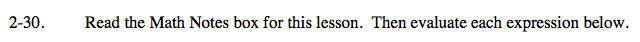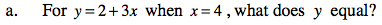Rewrite the expression, substituting in the known variable.

y = 2 + 3(4)
y = 2 + 12

y = 14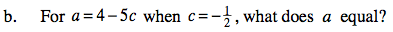See part (a) for a strategy to evaluate this expression.Remember to pay attention to negative signs.

a = 6.5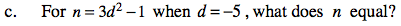Rewrite the expression, substituting in the known variable.

n = 3(−5)² − 1
n = 3(25) − 1
n = 75 − 1

n = 74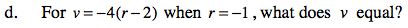Refer to parts (a) and (c).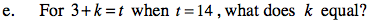Rewrite the problem, substituting in the known variable and then rearrange the problem to make it equal to the unknown variable.